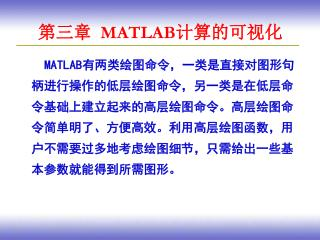DownloadDownload Presentation# 第三章 MATLAB 计算的可视化

Download Presentation## 第三章 MATLAB 计算的可视化

- - - - - - - - - - - - - - - - - - - - - - - - - - - E N D - - - - - - - - - - - - - - - - - - - - - - - - - - -
##### Presentation Transcript

1. 第三章 MATLAB计算的可视化 MATLAB有两类绘图命令，一类是直接对图形句柄进行操作的低层绘图命令，另一类是在低层命令基础上建立起来的高层绘图命令。高层绘图命令简单明了、方便高效。利用高层绘图函数，用户不需要过多地考虑绘图细节，只需给出一些基本参数就能得到所需图形。

2. 第三章 MATLAB计算的可视化 本章学习目标 ● 掌握绘制二维和三维图形的高层绘图函数。 ● 掌握图形控制函数的使用方法。 ● 了解图像处理的基本方法和动画制作的基本原理。

3. §3.1 二维曲线的绘制 3.1.1 绘制二维曲线 1．plot函数 plot(x,y) 其中x和y为大小相同的向量，分别用于存储x坐标和y坐标数据。 【例3.1】绘制曲线 程序： t=0:0.1:8*pi; x=cos(t)+t.*sin(t); y=sin(t)-t.*cos(t); plot(x,y);

4. §3.1 二维曲线的绘制 在实际应用中，plot函数的输入参数有许多变化形式： （1）当x和y是同维矩阵时，配对的x、y按对应列元素为横、纵坐标分别绘制曲线，曲线条数等于矩阵的列数。例如，在同一坐标系中绘制3条幅值不同的正弦曲线，命令为： x=0:pi/10:2*pi; y=sin(x); plot([x;x;x]',[y;y*2;y*3]')

5. §3.1 二维曲线的绘制 当x是向量，y是有一维与x同维的矩阵时，则绘制出多根曲线，曲线条数等于y矩阵的另一维数，x被作为这些曲线共同的横坐标。例如，在同一坐标中绘制3条幅值不同的正弦曲线，命令也可以写成： x=0:pi/10:2*pi; y=sin(x); plot(x,[y;y*2;y*3])

6. §3.1 二维曲线的绘制 （2）当plot函数只有一个输入参数时，即 plot(y) 若y是实型向量，则以该向量元素的下标为横坐标、元素值为纵坐标画出一条连续曲线；例如： y=rand(100,1); plot(y)

7. §3.1 二维曲线的绘制 DES密码算法初始置换表

8. §3.1 二维曲线的绘制 若y是复数向量，则分别以向量元素实部和虚部为横、纵坐标绘制一条曲线。 x=rand(100,1); y=rand(100,1); z=x+y.*i; plot(z)

9. §3.1 二维曲线的绘制 若y是实矩阵，则按列绘制每列元素值相对其下标的曲线，曲线条数等于输入参数矩阵的列数。例如： x=0:0.01*pi:pi; y=[sin(x’),cos(x’)]; plot([x’,x’],y)

10. §3.1 二维曲线的绘制 若y是复数矩阵，则按列分别以元素实部和虚部为横、纵坐标绘制多条曲线。 例如，绘制3个同心圆，命令为： t=0:0.01:2*pi; x=exp(i*t); y=[x;2*x;3*x]’; plot(y)

11. §3.1 二维曲线的绘制 （3）当plot函数有多个输入参数，且都为向量时，即 plot(x1,y1,x2,y2,…,xn,yn) 其中，x1和y1，x2和y2，……，xn和yn分别组成一组向量对，每一组向量对的长度可以不同。每一向量对可以绘制出一条曲线，这样可以在同一坐标系内绘制出多条曲线。例如，在同一坐标中绘制3条幅值不同的正弦曲线，命令也可以写成： x=0:pi/10:2*pi; y=sin(x); plot(x,y,x,y*2,x,y*3)

12. §3.1 二维曲线的绘制

13. §3.1 二维曲线的绘制 2．具有两个纵坐标标度的图形 绘制出具有不同纵坐标标度的两个图形，可以使用plotyy绘图函数。这种图形有利于图形数据的对比分析。 plotyy(x1,y1,x2,y2) 其中，x1和y1对应一条曲线，x2和y2对应另一条曲线。横坐标的标度相同，纵坐标有两个，左纵坐标用于x1、y1数据对，右纵坐标用于x2、y2数据对。

14. §3.1 二维曲线的绘制 【例3.2】用不同标度在同一坐标内绘制曲线y1=0.2e−0.5xcos(4x)和y2=1.5e−0.5xcos(x)。 程序如下： x=0:pi/100:2*pi; y1=0.2*exp(-0.5*x).*cos(4*pi*x); y2=1.5*exp(-0.5*x).*cos(pi*x); plotyy(x,y1,x,y2);

15. §3.1 二维曲线的绘制 plotyy函数还有其他两种调用格式如下： plotyy(x1,y1,x2,y2,fun); plotyy(x1,y1,x2,y2,fun1,fun2）; 其中，第2种调用格式是以fun方式绘制图形，fun可以为plot,semilogx,semilogy,loglog等；而第3种调用格式则是以fun1绘制（x1,y1），以fun2绘制（x2,y2).

16. §3.1 二维曲线的绘制 注意：在双坐标绘制图形的调用过程中，不能够像后面介绍的plot函数那样对曲线属性进行设置，如果要对曲线的线型、颜色以及数据点的标识加以控制，应使用后面介绍的句柄图形控制来完成。

17. §3.1 二维曲线的绘制 【例3.3】绘制双坐标二维图。 x=0:0.1*pi:2*pi; y=sin(x); z=exp(x); plotyy(x,y,x,z,'plot', 'semilogy')

18. §3.1 二维曲线的绘制 3.1.2 设置曲线样式 MATLAB提供了一些绘图选项，用于确定所绘曲线的线型、颜色和数据点标记符号。例如，“b-.”表示蓝色点画线，“y:d”表示黄色虚线并用菱形符标记数据点。当选项省略时，MATLAB规定，线型一律用实线，颜色将根据曲线的先后顺序依次采用表3.2给出的前7种颜色。

19. §3.1 二维曲线的绘制 表3.2 颜色选项 表3.1 线型选项

20. §3.1 二维曲线的绘制 表3.3 标记符号选项

21. §3.1 二维曲线的绘制 要设置曲线样式可以在plot函数中加绘图选项，其调用格式为 plot(x1,y1,选项1,x2,y2,选项2,…,xn,yn,选项n) 【例3.4】在同一坐标内，分别用不同线型和颜色绘制曲线y1=0.2e−0.5xcos(4x)和y2=1.5e−0.5xcos(x)。标记两曲线交叉点。

22. §3.1 二维曲线的绘制 程序如下： x=linspace(0,2*pi,1000); y1=0.2*exp(-0.5*x).*cos(4*pi*x); y2=1.5*exp(-0.5*x).*cos(pi*x); k=find(abs(y1-y2)<1e-2); %查找y1与y2相等点的下标 x1=x(k); %取y1与y2相等点的x坐标 y3=0.2*exp(-0.5*x1).*cos(4*pi*x1); %求y1与y2值相等点的y坐标 plot(x,y1,x,y2,'k:',x1,y3,'bp');

23. §3.1 二维曲线的绘制

24. §3.1 二维曲线的绘制 3.1.3 图形标注与坐标控制 1．图形标注 在绘制图形的同时，可以对图形加上一些说明，如图形名称、坐标轴说明、图形某一部分的含义等，这些操作称为添加图形标注。有关图形标注函数的调用格式如下。 ● title（图形名称） ● xlabel（x轴说明） ● ylabel（y轴说明） ● text（x，y，图形说明） ● legend（图例1，图例2，……）

25. §3.1 二维曲线的绘制 title和xlabel、ylabel 函数分别用于说明图形和坐标轴的名称。text函数是在（x，y）坐标处添加图形说明。添加文本说明也可用gtext命令，执行该命令时，十字坐标光标自动跟随鼠标移动，单击鼠标即可将文本放置在十字光标处，如命令gtext(‘cos(x)’)，即可放置字符串cos（x）。legend函数用于绘制曲线所用线型、颜色或数据点标记图例，图例放置在空白处，用户还可以通过鼠标移动图例，将其放置在所希望的位置。除legend函数外，其他函数同样适用于三维图形，z坐标轴说明用zlabel函数。

26. §3.1 二维曲线的绘制 上述函数中的说明文字，除使用标准的ASCII字符外，还可使用LaTeX（LaTeX是一种十分流行的数学排版软件）格式的控制字符，这样就可以在图形上添加希腊字母、数学符号、公式等内容。在MATLAB支持的LaTeX字符串中，用\bf、\it、\rm控制字符分别定义黑体、斜体和正体字符，受LaTeX字符串控制部分要加大括号{}括起来。 例如使得“MATLAB”一词黑体显示 text（0.3,0.5,‘The useful {\bf MATLAB}’） 得到标注效果sin(t+) text(0.3,0.5,'sin({\omega} t+{\beta})')

27. 表3.4 常用的LeTeX字符

28. §3.1 二维曲线的绘制 如果想在某个字符后面加上一个上标，则可以在该字符后面跟一个^引导字符串。若想把多个字符作为指数，则应该使用大括号，例如，e^{axt},对应的标注效果为 ，而e^axt对应的标注效果为 。类似的可以定义下标，下标是由-引导的，如 对应的标注效果为

29. §3.1 二维曲线的绘制 【例3.5】在0≤x≤2区间内，绘制曲线y1=e−0.5x和y2=cos(4x)e−0.5x，并添加图形标注。 程序如下： x=0:pi/100:2*pi; y1=exp(-0.5*x); y2=exp(-0.5*x).*sin(2*x); plot(x,y1,x,y2)

30. §3.1 二维曲线的绘制 title('x from 0 to 2{\pi}'); %加图形标题 xlabel('Variable X'); %加X轴说明 ylabel('Variable Y'); %加Y轴说明 text(1.5,0.5,'曲线y1=e^{-0.5x}'); %在指定位置添加图形说明 text(3,-0.1,'曲线y2=cos(4{\pi}x)e^{-0.5x}'); legend('y1','y2') %加图例

31. §3.1 二维曲线的绘制

32. §3.1 二维曲线的绘制 坐标轴标注的调用格式,以x轴为例再举一例： xlabel(‘标注’,‘属性1’，‘属性值1’，‘属性2’，‘属性值2’，…)

33. §3.1 二维曲线的绘制 【例3.6】坐标轴标注函数应用。 x=1:0.1*pi:2*pi; y=sin(x); plot(x,y) xlabel('x(0-2\pi)','FontWeight','bold') ylabel('y=sin(x)','FontWeight','bold') title('正弦函数','FontSize',12,'FontWeight','bold','FontName','隶书')

34. §3.1 二维曲线的绘制

35. §3.1 二维曲线的绘制 文本标注调用格式： text(x,y,’标注文本及控制字符串’) 【例3.7】对上例曲线添加文本。 text(3*pi/4,sin(3*pi/4),'\leftarrow sin(3\pi/4)=-0.707')

36. §3.1 二维曲线的绘制

37. §3.1 二维曲线的绘制 在标注中还可以实时地调用返回值为字符串的函数，如char,num2str等。利用这些函数可以完成较为复杂的文本标注。

38. §3.1 二维曲线的绘制 【例3.8】对上例曲线进行复杂的文本标注。 text(3*pi/4,sin(3*pi/4),['\leftarrow sin(3\pi/4)=',num2str(sin(3*pi/4))],'FontSize',20) text(5*pi/4,sin(5*pi/4),['sin(5\pi/4)=',num2str(sin(5*pi/4)),'\rightarrow'],...'HorizontalAlignment','right','FontSize',12)

39. §3.1 二维曲线的绘制 HorizontalAlignment,用来控制文本标识输入起点是在标识本身的左侧还是右侧

40. §3.1 二维曲线的绘制 交互式文本标注：gtext,使用该函数，可以通过鼠标来选择文本输入的点，单击后，系统将把指定的文本输入到所选的位置上。 【例3.9】鼠标交互式文本标注， x=1:0.1*pi:2*pi; y=sin(x); plot(x,y) gtext('y=sin(x)','FontSize',15)

41. §3.1 二维曲线的绘制

42. §3.1 二维曲线的绘制 图例标注：在数值计算结果的绘图中，经常会出现在同一张图形中绘制多条曲线的情况，为了能更好的区分各条曲线，matlab提供了图例标注函数legend。 其调用格式： legend(‘标注1’,’标注2’,…):标注1，标注2等分别对应绘图过程中按绘制先后顺序所生成的曲线。

43. §3.1 二维曲线的绘制 【例3.10】对多条曲线加图例标注。 x=0:0.1*pi:2*pi; y=sin(x); z=cos(x)； plot(x,y,x,z) legend('sin(x)','cos(x)')

44. §3.1 二维曲线的绘制

45. §3.1 二维曲线的绘制 对于图例框在图中的位置，有两种方式可以改变： 一、用鼠标拖动图例框改变其在图中的显示位置； 二、在legend函数调用中进行简单的定位设置。 legend函数的调用格式： legend(‘标注’,…,’定位代码’)

46. §3.1 二维曲线的绘制 matlab提供了6个定位代码： 0：自动定位，使得图标与图形重复最少； 1：置于图形的右上角（默认值）； 2：置于图形的左上角； 3：置于图形的左下角； 4：置于图形的右下角； -1：置于图形的右外侧。

47. §3.1 二维曲线的绘制 以上例的图像为例，进行图例框位置的设置，如下图：

48. §3.1 二维曲线的绘制 2．图形控制 matlab提供了较常用的图形控制函数，更加精细的控制由后面介绍的句柄图形来完成。 • 坐标轴的控制函数axis 函数axis用来控制坐标轴的刻度范围及显示形式。其调用格式如下： ●axis(V):其中v是一数组，用以存储坐标轴的范围，对于二维图形，v的表达形式为： v=[xmin,xmax,ymin,ymax] 对于三维图形，其表达形式为： V=[xmin,xmax,ymin,ymax,zmin,zmax]

49. §3.1 二维曲线的绘制 ●axis‘控制字符串’：使用这种格式用户可以选择不同的控制字符串，以完成对坐标轴的操作，具体的控制字符串的表达式形式如下页表：

50. axis控制字符串及说明